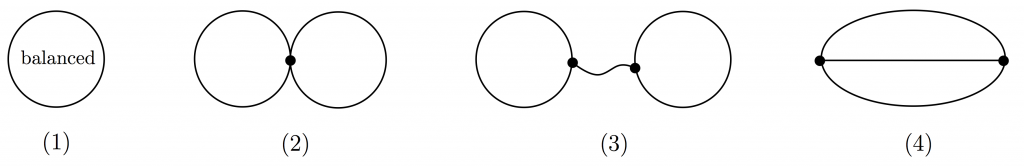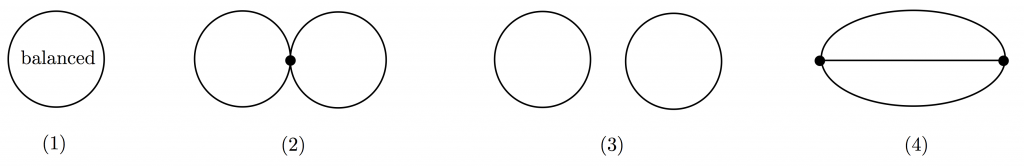# Biased graphs and their matroids I

In this post I will define some matroids on graphs and give some examples. Such examples include graphic matroids, bicircular matroids and Dowling geometries. These matroids arise in different contexts and this led to a profusion (and confusion) of terminology. As a consequence, it’s really difficult to look up literature and see what has or hasn’t been done in the area. I’ll try to give a road map of the terminology and the main results. For now I’ll mostly give basic definitions and examples, leaving results for the next post.

1. Biased graphs

All graphs in this post are allowed parallel edges and loops. I’ll constantly talk about cycles of a graph: as is common in graph theory, by a cycle I mean a nonempty connected subgraph in which every vertex has degree two. In various contexts these are also called polygons, circuits, circles… I’ll stick to the word “cycle”. I’ll also abuse terminology and use the word “cycle” to mean the set of edges of a cycle. By a nonempty path I mean a path with at least one edge. Let’s start by defining our general universe.

Def. biased graph is a pair $(G,\mathcal{B})$ where $\mathcal{B}$ is a set of cycles of $G$ satisfying the following property:

Theta property: for every two cycles $C_1$ and $C_2$ in $\mathcal{B}$ intersecting in a nonempty path, the third cycle in $C_1 \cup C_2$ is also in $\mathcal{B}$.

The cycles in $\mathcal{B}$ are called balanced, and the other cycles are unbalanced. Two cycles sharing a non-empty path form a theta; a theta with all cycles unbalanced is an unbalanced theta.

The theta property guarantees that the matroids defined in the next two sections are indeed matroids. In particular, this property guarantees that the third circuit axiom is satisfied.

Here are some important examples of biased graphs (the importance will become clear in the next sections):

• Biased graphs having only balanced cycles.
• Biased graphs having only unbalanced cycles.
• Signed graphs: consider a graph $G$ and a set of edges $F$ of $G$. Then we declare a cycle of $G$ to be balanced if it contains an even number of edges in $F$, and unbalanced otherwise. The resulting biased graph is called a signed graph. Balanced cycles in signed graphs are also called even or positive in the literature, while unbalanced cycles are also called odd or negative.
• Group labelled graphs: consider a graph $G$ and a (say multiplicative) group $\Gamma$. Orient every edge of $G$ and assign to every oriented edge a value from $\Gamma$. This is how we obtain group labelled graphs. Group labelled graphs are also called gain graphs or voltage graphs. From a group labelled graph we obtain a biased graph as follows. Pick any cycle $C$ of $G$ and choose an orientation for $C$. Traverse $C$ with this orientation and multiply the values on the traversed edges, where backward edges will get the inverse value (so if $(u,v)$ is an oriented edge with value $g$ and this edge appears as $(v,u)$ in the orientation of $C$, the value for this edge will be $g^{-1}$). If the total value along the cycle is $1$, then the cycle is declared to be balanced, otherwise it is unbalanced. Changing the orientation of a cycle only changes the value to its inverse, so this assignment is well defined. It is easy to see that this assignment of balanced/unbalanced cycles satisfies the theta property, so we obtain a biased graph from our group labelled graph. I’ll often abuse terminology and call this biased graph a group labelled graph. Signed graphs are special cases of group labelled graphs, obtained by labelling the edges of a graph with elements from $GF(2)$ or, equivalently, from the multiplicative group of the ternary field. I set them aside because they are relevant on their own.

Following are the two main matroids arising from biased graphs. This general setting was first introduced by Zaslavsky (see [Zas89] and [Zas91]).

2. Frame matroids

Def. Given a biased graph $\Omega=(G,\mathcal{B})$, the frame matroid represented by $\Omega$ has as elements the edges of $G$ and as circuits the edge sets of subgraphs of the following forms (see the figure below):

1. A balanced cycle.
2. Two unbalanced cycles sharing a vertex.
3. Two vertex-disjoint unbalanced cycles together with a minimal path joining them.
4. An unbalanced theta.Circuits of frame matroids are subdivisions of these graphs. Unlabelled cycles are unbalanced.

The circuits in 2. and 3. are called handcuffs (respectively tight and loose).

Zaslavsky [Zas91] calls these matroids biased matroids, but recently the term “frame matroids” has become more common. Originally, a frame matroid was defined to be a matroid $M$ to which a basis $B$ could be added so that every element of $M$ is spanned by two elements in $B$. Zaslavsky showed that this definition is equivalent to the one coming from biased graphs. Consider a frame matroid $M$ represented by a biased graph $\Omega$. Form a new biased graph $\Omega’$ by adding an unbalanced loop to every vertex of $\Omega$ and call $B$ the set of these unbalanced loops. Then $B$ is a basis of the frame matroid $M’$ of $\Omega’$ and $M$ is a restriction of $M’$. Every loop of $\Omega$ is either balanced (hence spanned by the empty set), or unbalanced (so in parallel to the element of $B$ incident to the same vertex). Moreover, every edge of $\Omega$ that is not a loop is spanned by the two loops of $B$ at its endpoints.

It follows immediately from the definition that the independent sets of the frame matroid of $(G,\mathcal{B})$ are the set of edges $F$ such that every component induced by $F$ contains at most one cycle, which will have to be unbalanced.

Here are some examples of frame matroids, arising from different choices for the biased graph $\Omega=(G,\mathcal{B})$.

• Graphic matroids: those are simply the frame matroids of biased graph with all cycles balanced. So frame matroids are a generalization of graphic matroids.
• Bicircular matroids: these are the frame matroids of biased graphs with all cycles unbalanced. Bicircular matroids have been widely studied (more of this in the next post, else no reader will make it to the definition of lift matroids).
• Signed-graphic matroids: these are frame matroids of signed graphs.
• Dowling geometries: first defined by Dowling ([Dow73]) these are frame matroids of group labelled graphs.

If $\mathbb{F}$ is a field and $\Omega$ is a group labelled graph on the multiplicative group of $\mathbb{F}$, then the frame matroid of $\Omega$ is $\mathbb{F}$-representable. A matrix representation is obtained in the following way: let $e$ be an edge of $\Omega$ with label $g$. If $e$ is a balanced loop (i.e. a loop labelled $1$), then the column for $e$ is an all-zero column; if $e$ is an unbalanced loop incident to vertex $u$, then the column for $e$ has the $u$ entry equal to $g$ and zero everywhere else.  If $e=uv$ is not a loop and $e$ is oriented as (u,v), then the corresponding column for $e$ has a $1$ in position $u$, $-g$ in position $v$ and zero everywhere else. The proof that this is a representation of the frame matroid of $\Omega$ is not obvious, but appears, for example, in [Zas82].

The class of frame matroids (and the subclasses defined above) are minor-closed classes. The same is true for lift matroids, defined in the next section. However, this post is long enough without adding matroid operations for biased graphs. I’ll leave that for the next post as well.

3. Lift matroids

Def. Given a biased graph $\Omega=(G,\mathcal{B})$, the lift matroid represented by $\Omega$ has as elements the edges of $G$ and as circuits the edge sets of subgraphs of the following forms (see the figure below):

1. A balanced cycle.
2. Two unbalanced cycles sharing a vertex.
3. Two vertex-disjoint unbalanced cycles.
4. An unbalanced theta.Circuits of lift matroids are subdivisions of these graphs. Unlabelled cycles are unbalanced.

At a first glance lift matroids may look quite similar to frame matroids, but the differences between them emerge as soon as you start working with them. My rule of thumb is that frame matroids are easier to deal with than lift matroids, for some definition of “easier”. Having circuits that are not connected in the graph makes things a lot more complicated in a lot of cases.

From the definition we can easily see that the independent sets of the lift matroid of $(G,\mathcal{B})$ are the set of edges $F$ containing at most one cycle, which will have to be unbalanced.

Here are some examples of lift matroids, arising from different choices for the biased graph $\Omega=(G,\mathcal{B})$.

• Graphic matroids: those are simply the lift matroids of biased graph with all cycles balanced. So lift matroids are also a generalization of graphic matroids.
• Even cycle matroids: these are lift matroids of signed graphs. Even cycle matroids appear in alternative proofs of the list of excluded minors for graphic matroids ([Ger95]) and Seymour’s decomposition of regular matroids ([GG95]). In fact, any binary single element coextension of a graphic matroid is an even cycle matroid.
• Spikes: let $G$ be obtained from the cycle on $n$ edges by doubling every edge. Declare every pair of parallel edges to form an unbalanced cycle. The other cycles of the graph may be declared to be balanced or unbalanced, as long as the theta property is satisfied. The lift matroid of the resulting biased graph is a spike with no tip. To obtain a spike with a tip, add an unbalanced loop to the graph.

If $\mathbb{F}$ is a field and $\Omega$ is a group labelled graph on the additive group of $\mathbb{F}$, then the frame matroid of $\Omega$ is $\mathbb{F}$-representable. A matrix representation is obtained in the following way: let $A$ be the vertex-edge oriented incidence matrix of $G$, with the orientation given in the group labelled graph. Add a row to $A$, where the entry for edge $e$ is the group label of $e$. The reader is invited to check that this is a valid matrix representation for the matroid.

In the next post I’ll survey some problems and results regarding these matroids.

References

[Dow73] T.A. Dowling, A class of geometric lattices based on finite groups, J. Combin. Theory Ser. B 14 (1973), 61–86.

[GG05] J. Geelen, A.M.H. Gerards, Regular matroid decomposition via signed graphs, J. Graph Theory 48 (2005), 74-84.

[Ger95] A.M.H. Gerards, On Tutte’s characterization of graphic matroids – a graphic proof, J. Graph Theory 20 (1995), 351-359.

[Zas82] T. Zaslavsky, Signed graphs, Discrete Applied Mathematics. 4 (1982), 47-74.

[Zas89] T. Zaslavsky, Biased graphs. I. Bias, balance, and gains, J. Combin. Theory Ser. B 47 (1989), 32-52.

[Zas91] T. Zaslavsky, Biased graphs. II. The three matroids, J. Combin. Theory Ser. B 51 (1991), 46-72.

## 3 thoughts on “Biased graphs and their matroids I”

1. Thanks for this excellent introduction to an important class of matroids. A small point that might mislead some readers: an unbalanced theta is usually defined to contain three unbalanced cycles. Likewise, in the definitions of circuits of the two matroids, the theta is not allowed to have any balanced cycle (otherwise it is not a minimal dependent set). Maybe you can change that?

•Irene Pivotto on said:

Thanks Stefan, you’re exactly right. The mistake should be fixed everywhere now.

This site uses Akismet to reduce spam. Learn how your comment data is processed.